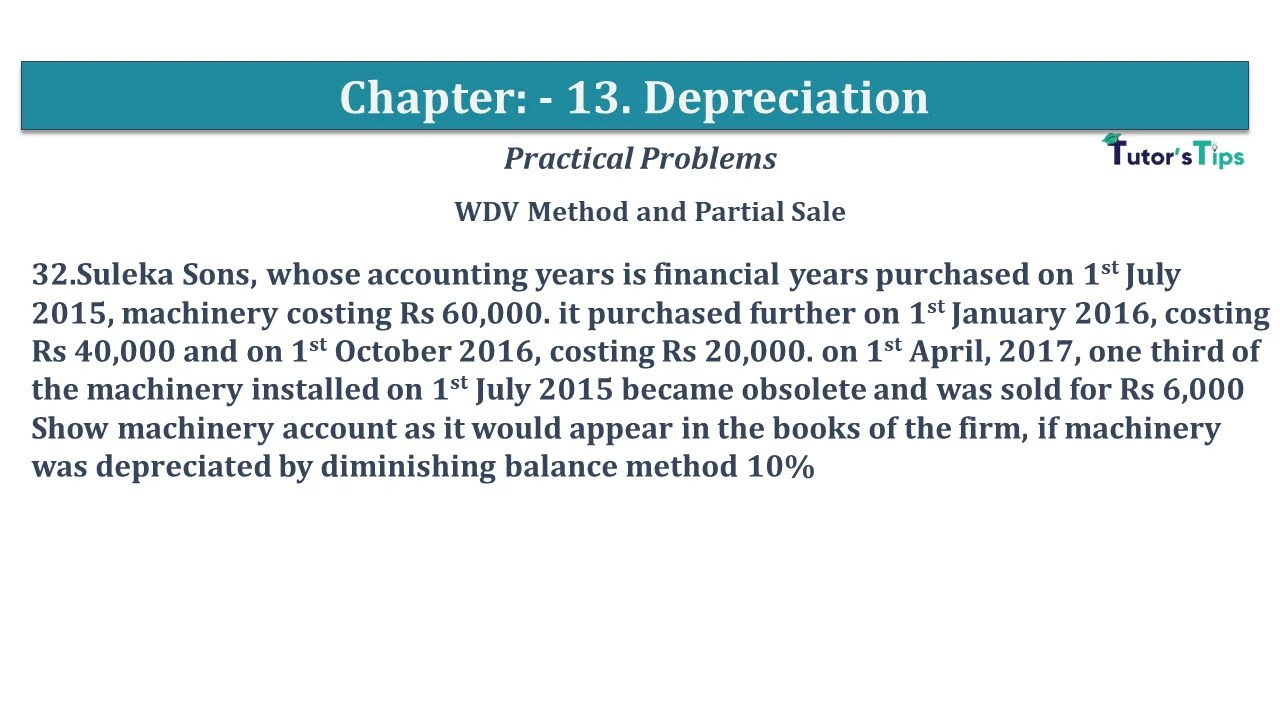# Question No 32 Chapter No 13 – USHA Publication 11 ClassQuestion No 32 Chapter No 13

WDV Method and Partial Sale

32.Suleka Sons, whose accounting years is financial years purchased on 1st July 2015, machinery costing Rs 60,000. it purchased further on 1st January 2016, costing Rs 40,000 and on 1st October 2016, costing Rs 20,000. on 1st April 2017, one-third of the machinery installed on 1st July 2015 became obsolete and was sold for Rs 6,000
Show machinery account as it would appear in the books of the firm if the machinery was depreciated by diminishing balance method 10%

The solution of Question No 32 Chapter No 13:-

 Dr. Machinery A/c Cr. Date Particulars J.F. Amount Date Particulars J.F. Amount 01/07/15 To Cash A/c 60,000 31/03/16 By Deprecation A/c*1 5,500 01/01/16 To Bank A/c 40,000 31/03/16 By Balance C/d 94,500 1,00,000 1,00,000 01/04/16 To Balance b/d 94,500 31/03/17 By Depreciation A/c*2 10,450 01/07/16 To Bank A/c 20,000 31/03/17 By Balance C/d 1,04,050 1,14,500 1,14,500 01/04/17 To Balance b/d 1,04,050 01/04/17 By Bank A/c 6,000 01/04/17 By Profit/Loss A/c 10,650 31/03/18 By Depreciation A/c*3 8,740 31/03/18 By Balance C/d 78,660 1,04,050 1,04,050

Working note:-

*1:- Calculation of the amount of Depreciation on furniture for the year 2015-16
Machinery purchased on 1st Jul 2015
Depreciation = Value of Asset X Rate of Depreciation X Period
Value of Asset = 60,000
Rate of Depreciation = 10%
Period = from 01/07/2015 to 31/03/2016 i.e. 9 months
(from the date of purchase/Beginning balance to end of the financial year)
= 60,000 X 10/100 X 9/ 12
Depreciation = 4,500
Machinery purchased on 1st Jan 2015
Depreciation = Value of Asset X Rate of Depreciation X Period
Value of Asset = 40,000
Rate of Depreciation = 10%
Period = from 01/10/2015 to 31/03/2016 i.e. 6 months
(from the date of purchase/Beginning balance to end of the financial year)
= 40,000 X 10/100 X 3/ 12
Depreciation = 1,000
Total Depreciation for the year = 5,500

*2:- Calculation of the amount of Depreciation on furniture for the year 2017-18
Machinery purchased on 1st Jul 2015
Depreciation = Value of Asset X Rate of Depreciation X Period
Value of Asset = 55,500
Rate of Depreciation = 10%
Period = from 01/04/2016 to 31/03/2017  i.e. 12 months
(from the date of purchase/Beginning balance to the end of the financial year)
= 55,500 X 10/100 X 12/ 12
Depreciation = 5,550
Machinery purchased on 1st Jan 2015
Depreciation = Value of Asset X Rate of Depreciation X Period
Value of Asset = 39,000
Rate of Depreciation = 10%
Period = from 01/12/2016 to 31/03/2017 i.e. 12 months
(from the date of purchase/Beginning balance to the end of the financial year)
= 39,000 X 10/100 X 12/ 12
Depreciation = 3,900
Machinery purchased on 1st Oct 2016
Depreciation = Value of Asset X Rate of Depreciation X Period
Value of Asset = 20,000
Rate of Depreciation = 10%
Period = from 01/10/2016 to 31/03/2017 i.e. 6 months
(from the date of purchase/Beginning balance to end of the financial year)
= 20,000 X 10/100 X 6/ 12
Depreciation = 1,000
Total Depreciation for the year = 10,450

 Statement Showing profit or loss on the sale of Machinery Particulars Amount Purchase value of machinery as on 1st July 2015 1/3 20,000 Less: – Amount of Depreciation charged on the year 2015-16 20,000 *10%* 9/12 1,500 Amount of Depreciation charged on the year 2016-17 18,500 *10%* 12/12 1,850 Book value of the asset as on 1st April 2017 16,650 Sale Price of Machinery 6,000 Profit on the sale of the asset 10,650

*3:- Calculation of the amount of Depreciation on furniture for the year 2018-19
Machinery purchased on 1st Jul 2015 ½
Depreciation = Value of Asset X Rate of Depreciation X Period
Value of Asset = 33,300
Rate of Depreciation = 10%
Period = from 01/04/2018 to 31/03/2019 i.e. 12 months
(from the date of purchase/Beginning balance to the end of the financial year)
= 33,300 X 10/100 X 12/ 12
Depreciation = 3,330
Machinery purchased on 1st Jan 2015
Depreciation = Value of Asset X Rate of Depreciation X Period
Value of Asset = 35,100
Rate of Depreciation = 10%
Period = from 01/04/2017 to 31/03/2018 i.e. 12 months
(from the date of purchase/Beginning balance to end of the financial year)
= 35,100 X 10/100 X 12/ 12
Depreciation = 3,510
Machinery purchased on 1st Oct 2016
Depreciation = Value of Asset X Rate of Depreciation X Period
Value of Asset = 19,000
Rate of Depreciation = 10%
Period = from 01/10/2017 to 31/03/2018 i.e. 12 months
(from the date of purchase/Beginning balance to end of the financial year)
= 20,000 X 10/100 X 6/ 12
Depreciation = 1,900
Total Depreciation for the year = 8,740

Depreciation | Meaning | Methods | Examples

Comment if you have any question.

Also, Check out the solved question of all Chapters: –

Chapter No. 1 – Introduction

Chapter No. 2 – Theory Base of Accounting

Chapter No. 3 – Vouchers and transactions

Chapter No. 4 – Journal

Chapter No. 5 – Ledger

Chapter No. 6 – Cash Book

Chapter No. 7 – Other Subsidiary Books

Chapter No. 8 – Journal Proper

Chapter No. 9 – Trial Balance

Chapter No. 10 – Bank Reconciliation Statement

Chapter No. 11 – Depreciation

Chapter No. 12 – Provisions and Reserves

Chapter No. 13 – Bills of Exchange

Chapter No. 14 – Rectification of Errors

Chapter No. 15 – Financial Statements – (Without Adjustments)

Chapter No. 16 – Financial Statements – (With Adjustments)